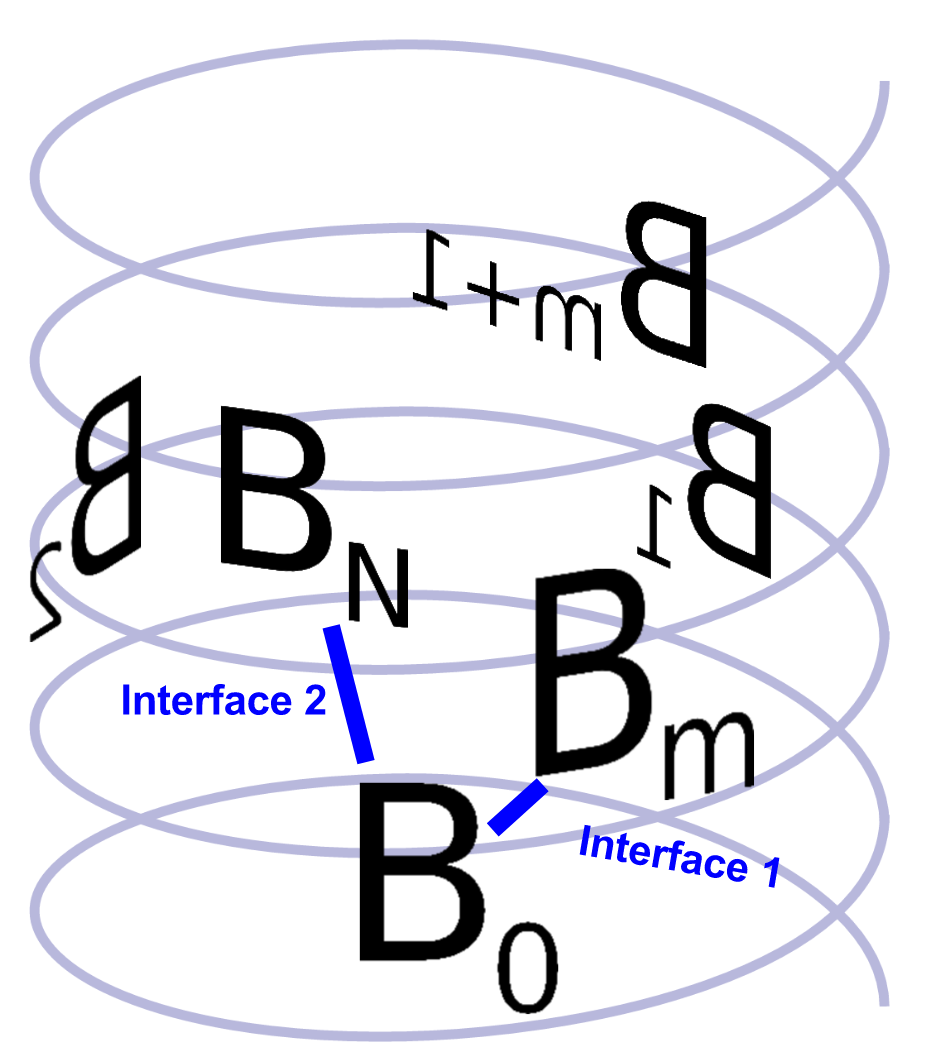# Examples¶

## high level¶

### fiber docking¶

This is prototype code to dock a monomer into helical protein fiber. Xform from $$B_0$$ to $$B_N$$ is sampled via hierarchical search. Possible placements a third body $$B_M$$ are checked based on possible $$M/N$$ roots of the transform $$X_N$$:

$X_M = X_N^{M/N}$"""
a prototype module for fiber docking. for illustration see:

(see prev slide for simpler, less-general case)
"""

# from rif.nest import NestedXforms
# from rif.models import ImplicitEnsemble, ImplicitEnsembleScore
# from rif.search import HierBeamSearch

# import numpy as np

def rel_prime_flat_tri(Nmax):
"""return two same-size lists of relatively prime numbers

mapped to tuples, would be: 1,2 1,3 2,3 1,4 3,4 1,5 2,5 3,5 4,5 ...
replace with numpy one-liner, probably more clear
"""
pass

def center_welzl(model):
"""center model based on welzl optimal bounding sphere, report radius"""
pass

def fiber_dock(pdb_file):
"""turn monomer into helical fiber in "all" possible ways"""

# setup
resolutions = (16, 8, 4, 2, 1, 0.5)
M, N = rel_prime_flat_tri(Nmax=20)
powers = (M / N)[np.newaxis, :]  # shape (1, whatever)
assert all(powers < 1)  # else Hsearch can't work
bbframes = prot.bbframes(ss='HE', burial='exposed')
# ImplicitEnsemble represents a structural ensemble in which each member's
# elements (atoms, rays, stubs, etc) are within well-defined translational
# and rotational bounds of a reference structure. (rotation mag defined by
ensemble0 = ImplicitEnsemble(atoms=prot.atoms, rotslots=bbframes,
hsearch = HierBeamSearch(resls=resolutions, grid=samp_grid, beam_size_m=10,
extra_fields=(('M', 'u1'), ('N', 'u1')), )
score = ImplicitEnsembleScore(
# todo
)

# assuming I can do what I want with numpy dtype/operators...
def score_samples(stage, samples):
resl = resolutions[stage]
indices = samples['index']
positions = samp_grid.position_of(stage, indices)
score0N = score(ensemble0, ensembleN)  # radius 0 + resl
# better to extract sub-array of score0N < 0?
if np.any(score0N < 0):
# in addition to ensemble['position']**(M/N), operator
# linearly by pow... is this well-defined algebra?
# maybe just use an ensemble_power() func directly?
ensembleM = np.power(ensembleN, powers)
# radius 0 + resl * m / n (not constant over array)
score0M = score(ensemble0, ensembleM)
# - coupling='linear' means M ensemble motion tied exactly to
# - this check may not be worth the cost here, skip or
#   maybe filter on ensembleM good enough first
scoreMN = score(ensembleM, ensembleN, coupling='linear')
score0MN = score0N + score0M + scoreMN  # score0N bcast
idxmin = score0MN.argmin(axis=2)  # best score forall M / N
samples['M'] = M[idxmin]
samples['N'] = N[idxmin]
samples['score'] = score0MN[:, idxmin]
# todo: eventually check all good M/N? will probably be rare
#       to have more than one hit
# todo: also score ensemble0 vs all 1..N) for thoroughness
return samples

# hsearch interface option 1
# simple, but perhaps not as flexible as below
hsearch.search(score_samples)
print(hsearch.results())

# hsearch interface option 2
# not sure if using iteration as an interface to the search is a
# good idea or not... note that samples['score'] gets communicated
# back to the search, which is not a pattern I've seen used much
# but it would be clearer for simpler cases... don't actually have to
# define a function (ie score_samples)
hsearch_accum = hsearch.accumulator()
for iresl, samples in hsearch_accum:
score_samples(iresl, samples)
print(hsearch_accum.results())

if __name__ == '__main__':
fiber_dock()